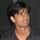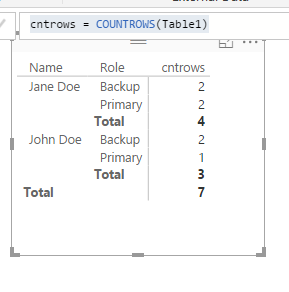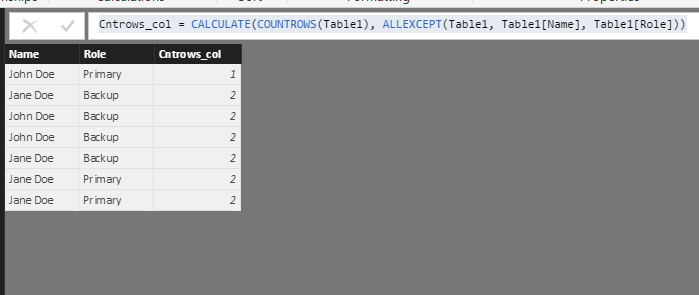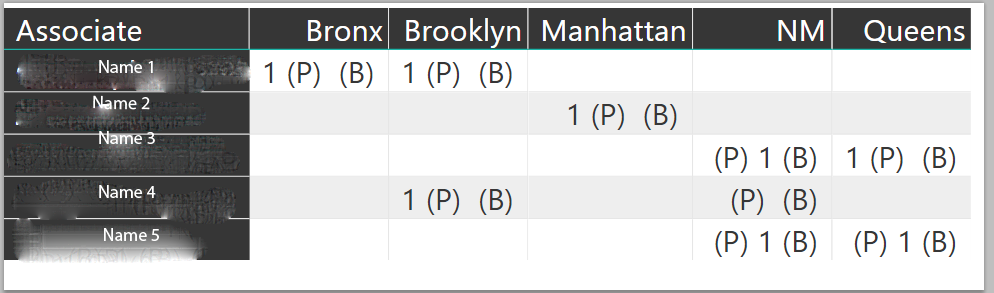cancel
Showing results for
Did you mean:Frequent Visitor

## Problem With Countrows Function

I'm strugling with DAX, I have been trying to create a New Column that counts the number of times a User in my database has a certain Role.

I have been using unsucessfully trying CALCULATE(COUNTROWS(FILTER('Contact role','Contact role'[Level]="Primary")))

I think I may be using the wrong functions for this, but have been unable to find a solution. Any help is greatly appreciated.

For more structure, here is the fake subset of the table named 'Contact role':

 Name Role John Doe Primary Jane Doe Backup John Doe Backup John Doe Backup Jane Doe Backup Jane Doe Primary Jane Doe Primary

1 ACCEPTED SOLUTIONMemorable Member

I just used your formula in a measure (and not a calculated column), and then used it on our sample data. It seems to give the right results.

`cntrows = CALCULATE(COUNTROWS(Table1), FILTER(Table1, Table1[Role]="Primary")) & " P  " &  CALCULATE(COUNTROWS(Table1), FILTER(Table1, Table1[Role]="Backup")) & " B"`It would be easier for us to help you if you give sample data (like you gave), and then show exactly what the results should be with the sample data. Feel free to change the sample data but make sure that you show what the end results also need to be, so that we can replicate it in Power BI

4 REPLIES 4Memorable Member

Can you tell us what the output needs to be?

If you make a table with the name, role and a simple countrows measure

`cntrows = COUNTROWS(Table1)`

You will get the following outputIf you want it as a caclulated column (maybe as an intermediate step for some other calculation), you can use the formula below

`Cntrows_col = CALCULATE(COUNTROWS(Table1), ALLEXCEPT(Table1, Table1[Name], Table1[Role]))`So depends on what your use is.Frequent Visitor

I'm trying to make it so I can show Both on a single table

I censored the table I am trying to create. The Associate is the name, and the role is relative to the Borough of New York City.

The formula I used for this is CALCULATE(COUNTROWS(FILTER('Contact role','Contact role'[Level]="Primary")))&" (P) "&CALCULATE(COUNTROWS(FILTER('Contact role','Contact role'[Level]="Backup")))&" (B) "Memorable Member

I just used your formula in a measure (and not a calculated column), and then used it on our sample data. It seems to give the right results.

`cntrows = CALCULATE(COUNTROWS(Table1), FILTER(Table1, Table1[Role]="Primary")) & " P  " &  CALCULATE(COUNTROWS(Table1), FILTER(Table1, Table1[Role]="Backup")) & " B"`It would be easier for us to help you if you give sample data (like you gave), and then show exactly what the results should be with the sample data. Feel free to change the sample data but make sure that you show what the end results also need to be, so that we can replicate it in Power BIFrequent Visitor

That solved my problem, thank you so much I can't believe it was that simple.

I'm sorry I couldn't provide the data, it was confidental (if you couldn't tell by my bad attempt to censor ha)

Thanks